DraftKings Championship Odds
+200
2 to 1Clemson
23.8% implied probability

+250
2.5 to 1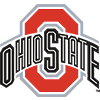Ohio State
20.4% implied probability

+400
4 to 1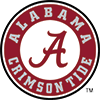Alabama
14.3% implied probability

+1200
12 to 1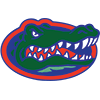Florida
5.5% implied probability

+2000
20 to 1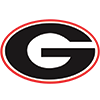Georgia
3.4% implied probability

+2800
28 to 1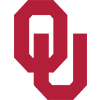Oklahoma
2.5% implied probability

+2800
28 to 1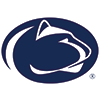Penn State
2.5% implied probability

+4000
40 to 1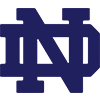Notre Dame
1.7% implied probability

+4000
40 to 1Texas
1.7% implied probability

+4000
40 to 1Wisconsin
1.7% implied probability

+4000
40 to 1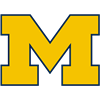Michigan
1.7% implied probability

+4000
40 to 1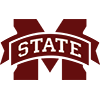Mississippi State
1.7% implied probability

+5000
50 to 1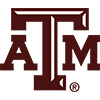Texas A&M
1.4% implied probability

+6600
66 to 1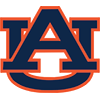Auburn
1.1% implied probability

+8000
80 to 1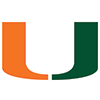Miami
0.9% implied probability

+10000
100 to 1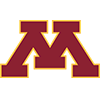Minnesota
0.7% implied probability

+10000
100 to 1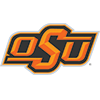Oklahoma State
0.7% implied probability

+10000
100 to 1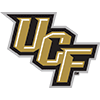Central Florida
0.7% implied probability

+10000
100 to 1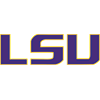LSU
0.7% implied probability

+10000
100 to 1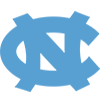North Carolina
0.7% implied probability

+10000
100 to 1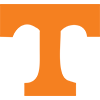Tennessee
0.7% implied probability

+12500
125 to 1Pittsburgh
0.6% implied probability

+15000
150 to 1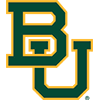Baylor
0.5% implied probability

+15000
150 to 1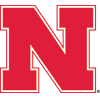Nebraska
0.5% implied probability

+15000
150 to 1Virginia Tech
0.5% implied probability

No changes have been recorded yet.
+15000
150 to 1Iowa
0.5% implied probability

+15000
150 to 1Memphis
0.5% implied probability

No changes have been recorded yet.
+20000
200 to 1Kansas State
0.4% implied probability

+20000
200 to 1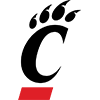Cincinnati
0.4% implied probability

+20000
200 to 1Boston College
0.4% implied probability

+25000
250 to 1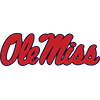Mississippi
0.3% implied probability

+25000
250 to 1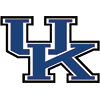Kentucky
0.3% implied probability

+25000
250 to 1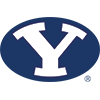BYU
0.3% implied probability

+25000
250 to 1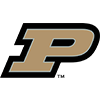Purdue
0.3% implied probability

+25000
250 to 1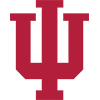Indiana
0.3% implied probability

+25000
250 to 1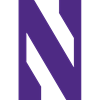Northwestern
0.3% implied probability

+25000
250 to 1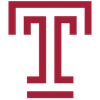Temple
0.3% implied probability

No changes have been recorded yet.
+25000
250 to 1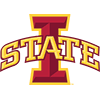Iowa State
0.3% implied probability

+25000
250 to 1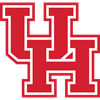Houston
0.3% implied probability

No changes have been recorded yet.
+30000
300 to 1Marshall
0.2% implied probability

+35000
350 to 1Virginia
0.2% implied probability

+35000
350 to 1Wake Forest
0.2% implied probability

+35000
350 to 1West Virginia
0.2% implied probability

+35000
350 to 1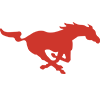SMU
0.2% implied probability

+35000
350 to 1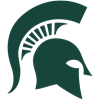Michigan State
0.2% implied probability

+50000
500 to 1Florida Atlantic
0.1% implied probability

No changes have been recorded yet.
+50000
500 to 1North Texas
0.1% implied probability

No changes have been recorded yet.
+50000
500 to 1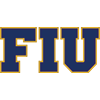Florida International
0.1% implied probability

No changes have been recorded yet.
+50000
500 to 1Navy
0.1% implied probability

+50000
500 to 1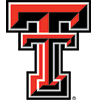Texas Tech
0.1% implied probability

+50000
500 to 1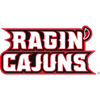Louisiana-Lafayette
0.1% implied probability

No changes have been recorded yet.
+50000
500 to 1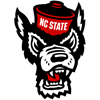North Carolina State
0.1% implied probability

+50000
500 to 1Army
0.1% implied probability

No changes have been recorded yet.
+50000
500 to 1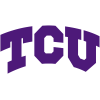TCU
0.1% implied probability

+50000
500 to 1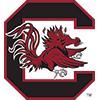South Carolina
0.1% implied probability

+50000
500 to 1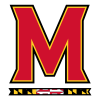Maryland
0.1% implied probability

+50000
500 to 1Missouri
0.1% implied probability

+100000
1000 to 1Georgia State
0.1% implied probability

No changes have been recorded yet.
+100000
1000 to 1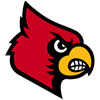Louisville
0.1% implied probability

+100000
1000 to 1Appalachian State
0.1% implied probability

No changes have been recorded yet.
+100000
1000 to 1Duke
0.1% implied probability

+100000
1000 to 1Vanderbilt
0.1% implied probability

No changes have been recorded yet.
+100000
1000 to 1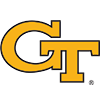Georgia Tech
0.1% implied probability

+100000
1000 to 1Western Kentucky
0.1% implied probability

No changes have been recorded yet.
+100000
1000 to 1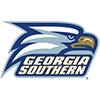Georgia Southern
0.1% implied probability

No changes have been recorded yet.
+100000
1000 to 1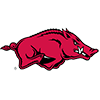Arkansas
0.1% implied probability

No changes have been recorded yet.
+100000
1000 to 1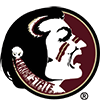Florida State
0.1% implied probability

+100000
1000 to 1Arkansas State
0.1% implied probability

No changes have been recorded yet.
+100000
1000 to 1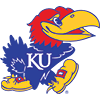Kansas
0.1% implied probability

+100000
1000 to 1UNC Charlotte
0.1% implied probability

No changes have been recorded yet.
+100000
1000 to 1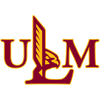Louisiana-Monroe
0.1% implied probability

No changes have been recorded yet.
+100000
1000 to 1Rice
0.1% implied probability

No changes have been recorded yet.
+100000
1000 to 1East Carolina
0.1% implied probability

+100000
1000 to 1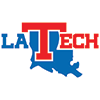Louisiana Tech
0.1% implied probability

No changes have been recorded yet.
+100000
1000 to 1South Alabama
0.1% implied probability

No changes have been recorded yet.
+100000
1000 to 1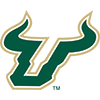South Florida
0.1% implied probability

+100000
1000 to 1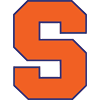Syracuse
0.1% implied probability

+100000
1000 to 1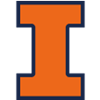Illinois
0.1% implied probability

+100000
1000 to 1Coastal Carolina
0.1% implied probability

No changes have been recorded yet.
+100000
1000 to 1Texas State
0.1% implied probability

No changes have been recorded yet.
+100000
1000 to 1Troy
0.1% implied probability

No changes have been recorded yet.
+100000
1000 to 1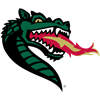UAB
0.1% implied probability

No changes have been recorded yet.
+100000
1000 to 1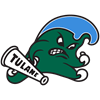Tulane
0.1% implied probability

+500000
5000 to 1Middle Tenn. St
0.0% implied probability

+500000
5000 to 1Southern Miss
0.0% implied probability

+500000
5000 to 1UTSA
0.0% implied probability

No changes have been recorded yet.
+500000
5000 to 1UTEP
0.0% implied probability

No changes have been recorded yet.